# 【预测模型-DELM分类】基于哈里斯鹰算法改进深度学习极限学习机实现数据分类附matlab代码

+关注继续查看

✅作者简介：热爱科研的Matlab仿真开发者，修心和技术同步精进，matlab项目合作可私信。

🍎个人主页：Matlab科研工作室

🍊个人信条：格物致知。

## ⛄ 内容介绍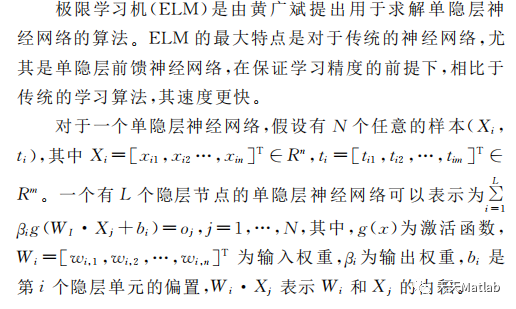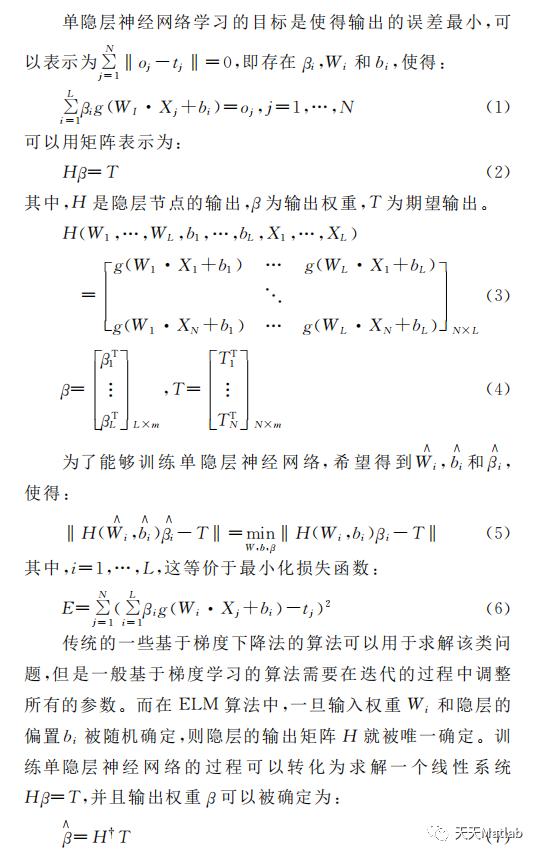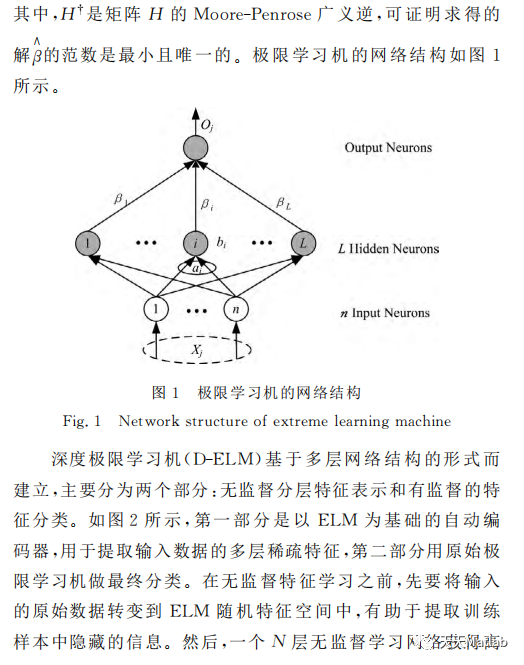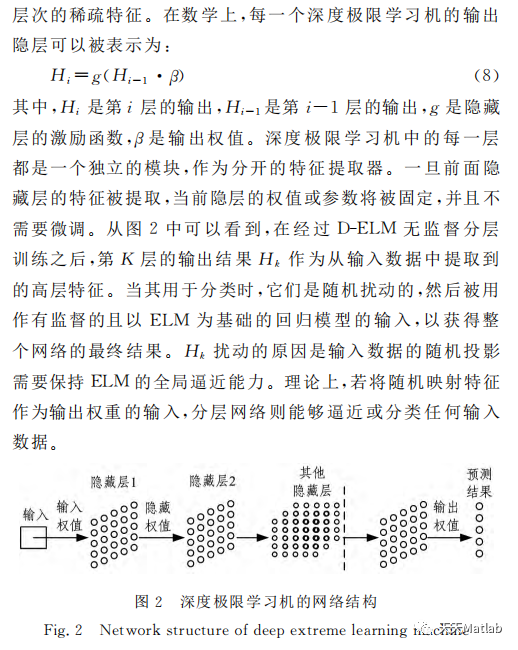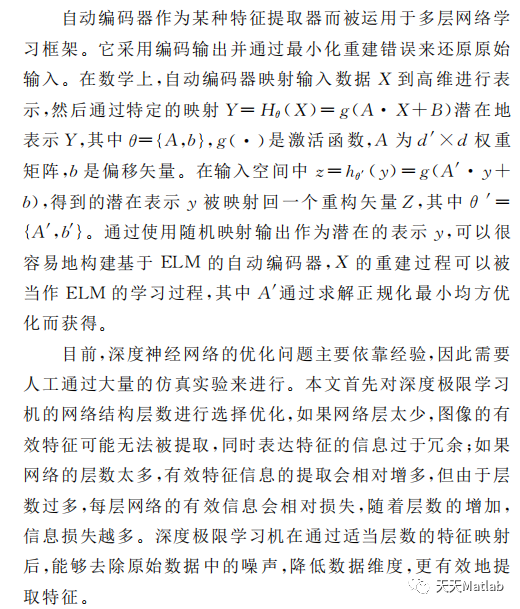## ⛄ 部分代码

% Developed in MATLAB R2013b

% Source codes demo version 1.0

% _____________________________________________________

% Main paper:

% Harris hawks optimization: Algorithm and applications

% Ali Asghar Heidari, Seyedali Mirjalili, Hossam Faris, Ibrahim Aljarah, Majdi Mafarja, Huiling Chen

% Future Generation Computer Systems,

% _____________________________________________________

% You can run the HHO code online at codeocean.com  https://doi.org/10.24433/CO.1455672.v1

% You can find the HHO code at https://github.com/aliasghar68/Harris-hawks-optimization-Algorithm-and-applications-.git

% _____________________________________________________

%  Author, inventor and programmer: Ali Asghar Heidari,

%  PhD research intern, Department of Computer Science, School of Computing, National University of Singapore, Singapore

%  Exceptionally Talented Ph. DC funded by Iran's National Elites Foundation (INEF), University of Tehran

%  03-03-2019

%  Researchgate: https://www.researchgate.net/profile/Ali_Asghar_Heidari

%  e-Mail: as_heidari@ut.ac.ir, aliasghar68@gmail.com,

%  e-Mail (Singapore): aliasgha@comp.nus.edu.sg, t0917038@u.nus.edu

% _____________________________________________________

%  Co-author and Advisor: Seyedali Mirjalili

%

%         e-Mail: ali.mirjalili@gmail.com

%                 seyedali.mirjalili@griffithuni.edu.au

%

%       Homepage: http://www.alimirjalili.com

% _____________________________________________________

%  Co-authors: Hossam Faris, Ibrahim Aljarah, Majdi Mafarja, and Hui-Ling Chen

%       Homepage: http://www.evo-ml.com/2019/03/02/hho/

% _____________________________________________________

%% %%%%%%%%%%%%%%%%%%%%%%%%%%%%%%%%%%%%%%%%%%%%%%%%%%%%%%%%%%%%%%%%%%

% Harris's hawk optimizer: In this algorithm, Harris' hawks try to catch the rabbit.

% T: maximum iterations, N: populatoin size, CNVG: Convergence curve

% To run HHO: [Rabbit_Energy,Rabbit_Location,CNVG]=HHO(N,T,lb,ub,dim,fobj)

function [Rabbit_Energy,Rabbit_Location,CNVG]=HHO(N,T,lb,ub,dim,fobj)

disp('HHO is now tackling your problem')

tic

% initialize the location and Energy of the rabbit

Rabbit_Location=zeros(1,dim);

Rabbit_Energy=inf;

%Initialize the locations of Harris' hawks

X=initialization(N,dim,ub,lb);

CNVG=zeros(1,T);

t=0; % Loop counter

while t<T

for i=1:size(X,1)

% Check boundries

FU=X(i,:)>ub;FL=X(i,:)<lb;X(i,:)=(X(i,:).*(~(FU+FL)))+ub.*FU+lb.*FL;

% fitness of locations

fitness=fobj(X(i,:));

% Update the location of Rabbit

if fitness<Rabbit_Energy

Rabbit_Energy=fitness;

Rabbit_Location=X(i,:);

end

end

E1=2*(1-(t/T)); % factor to show the decreaing energy of rabbit

% Update the location of Harris' hawks

for i=1:size(X,1)

E0=2*rand()-1; %-1<E0<1

Escaping_Energy=E1*(E0);  % escaping energy of rabbit

if abs(Escaping_Energy)>=1

%% Exploration:

% Harris' hawks perch randomly based on 2 strategy:

q=rand();

rand_Hawk_index = floor(N*rand()+1);

X_rand = X(rand_Hawk_index, :);

if q<0.5

% perch based on other family members

X(i,:)=X_rand-rand()*abs(X_rand-2*rand()*X(i,:));

elseif q>=0.5

% perch on a random tall tree (random site inside group's home range)

X(i,:)=(Rabbit_Location(1,:)-mean(X))-rand()*((ub-lb)*rand+lb);

end

elseif abs(Escaping_Energy)<1

%% Exploitation:

% Attacking the rabbit using 4 strategies regarding the behavior of the rabbit

%% phase 1: surprise pounce (seven kills)

% surprise pounce (seven kills): multiple, short rapid dives by different hawks

r=rand(); % probablity of each event

if r>=0.5 && abs(Escaping_Energy)<0.5 % Hard besiege

X(i,:)=(Rabbit_Location)-Escaping_Energy*abs(Rabbit_Location-X(i,:));

end

if r>=0.5 && abs(Escaping_Energy)>=0.5  % Soft besiege

Jump_strength=2*(1-rand()); % random jump strength of the rabbit

X(i,:)=(Rabbit_Location-X(i,:))-Escaping_Energy*abs(Jump_strength*Rabbit_Location-X(i,:));

end

%% phase 2: performing team rapid dives (leapfrog movements)

if r<0.5 && abs(Escaping_Energy)>=0.5, % Soft besiege % rabbit try to escape by many zigzag deceptive motions

Jump_strength=2*(1-rand());

X1=Rabbit_Location-Escaping_Energy*abs(Jump_strength*Rabbit_Location-X(i,:));

if fobj(X1)<fobj(X(i,:)) % improved move?

X(i,:)=X1;

else % hawks perform levy-based short rapid dives around the rabbit

X2=Rabbit_Location-Escaping_Energy*abs(Jump_strength*Rabbit_Location-X(i,:))+rand(1,dim).*Levy(dim);

if (fobj(X2)<fobj(X(i,:))), % improved move?

X(i,:)=X2;

end

end

end

if r<0.5 && abs(Escaping_Energy)<0.5, % Hard besiege % rabbit try to escape by many zigzag deceptive motions

% hawks try to decrease their average location with the rabbit

Jump_strength=2*(1-rand());

X1=Rabbit_Location-Escaping_Energy*abs(Jump_strength*Rabbit_Location-mean(X));

if fobj(X1)<fobj(X(i,:)) % improved move?

X(i,:)=X1;

else % Perform levy-based short rapid dives around the rabbit

X2=Rabbit_Location-Escaping_Energy*abs(Jump_strength*Rabbit_Location-mean(X))+rand(1,dim).*Levy(dim);

if (fobj(X2)<fobj(X(i,:))), % improved move?

X(i,:)=X2;

end

end

end

%%

end

end

t=t+1;

CNVG(t)=Rabbit_Energy;

%    Print the progress every 100 iterations

%    if mod(t,100)==0

%        display(['At iteration ', num2str(t), ' the best fitness is ', num2str(Rabbit_Energy)]);

%    end

end

toc

end

% ___________________________________

function o=Levy(d)

beta=1.5;

sigma=(gamma(1+beta)*sin(pi*beta/2)/(gamma((1+beta)/2)*beta*2^((beta-1)/2)))^(1/beta);

u=randn(1,d)*sigma;v=randn(1,d);step=u./abs(v).^(1/beta);

o=step;

end

## ⛄ 运行结果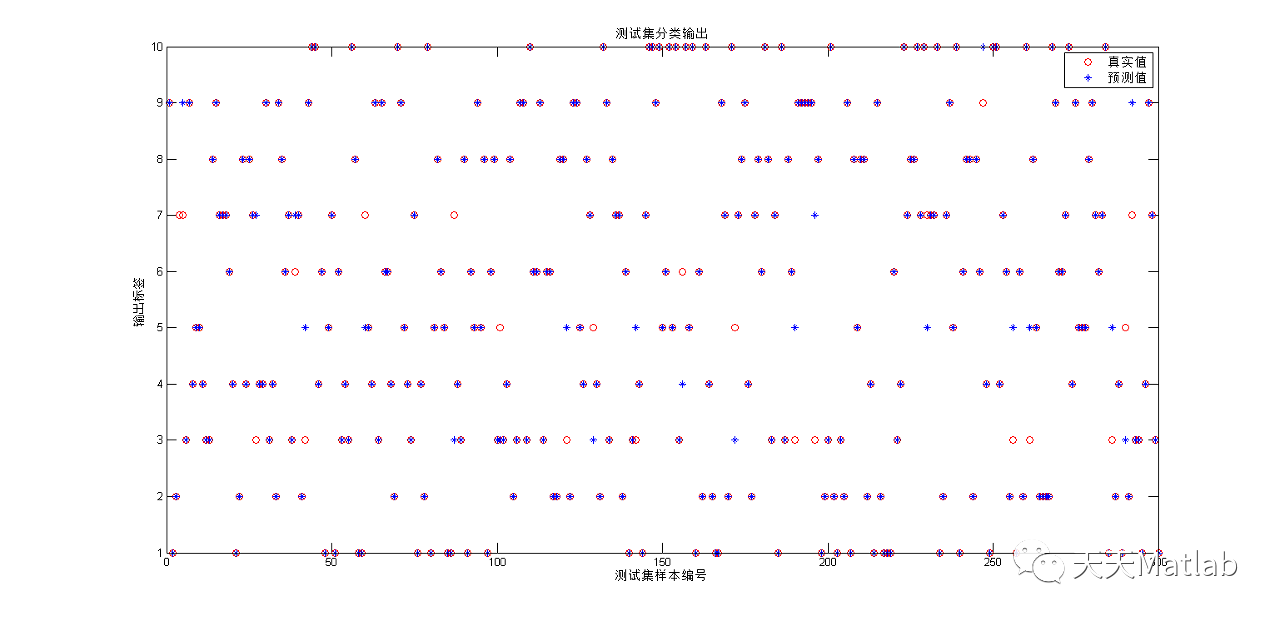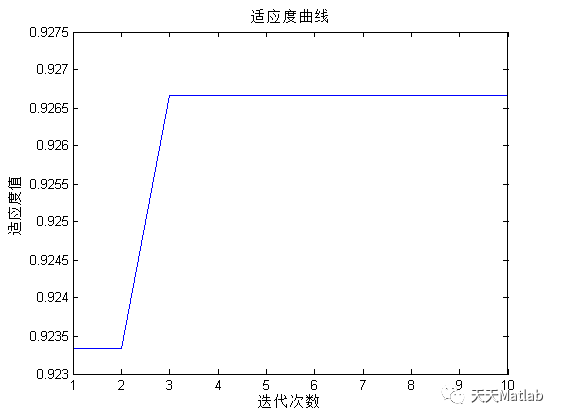## ⛄ 参考文献

吴丁杰, 温立书. 一种基于哈里斯鹰算法优化的核极限学习机[J]. 信息通信, 2021(034-011).

##### ❤️部分理论引用网络文献，若有侵权联系博主删除|
8月前
|

【pytorch深度学习实践】笔记—05.pytorch实现线性回归
【pytorch深度学习实践】笔记—05.pytorch实现线性回归
210 0
|
9月前
|

【DELM回归预测】基于海鸥算法改进深度学习极限学习机SOA-DELM实现数据回归预测附matlab代码
【DELM回归预测】基于海鸥算法改进深度学习极限学习机SOA-DELM实现数据回归预测附matlab代码
79 1
|
9月前
|

【DELM回归预测】基于粒子群算法改进深度学习极限学习机PSO-DELM实现数据回归预测附matlab代码
【DELM回归预测】基于粒子群算法改进深度学习极限学习机PSO-DELM实现数据回归预测附matlab代码
70 0
|
9月前
|

【DELM回归预测】基于灰狼算法改进深度学习极限学习机GWO-DELM实现数据回归预测附matlab代码
【DELM回归预测】基于灰狼算法改进深度学习极限学习机GWO-DELM实现数据回归预测附matlab代码
105 0
|
9月前
|

【DELM回归预测】基于人工蜂群算法改进深度学习极限学习机ABC-DELM实现数据回归预测附matlab代码
【DELM回归预测】基于人工蜂群算法改进深度学习极限学习机ABC-DELM实现数据回归预测附matlab代码
97 0